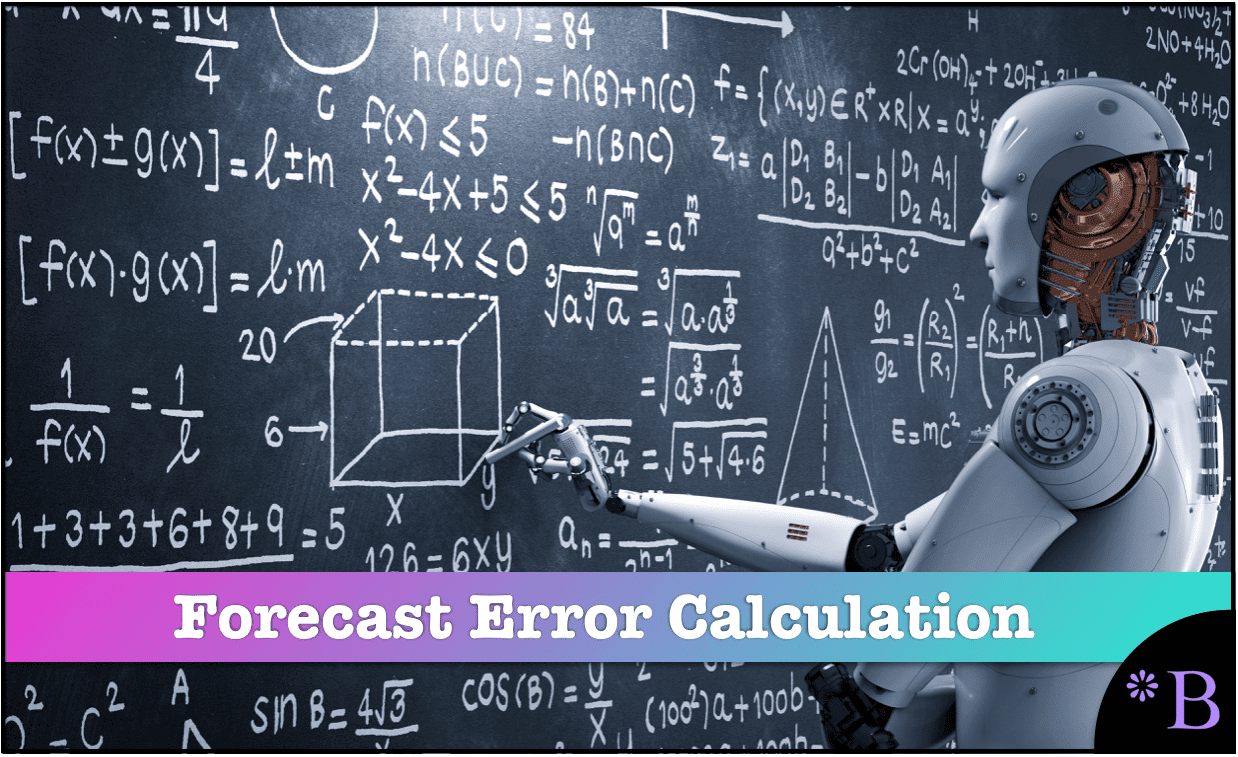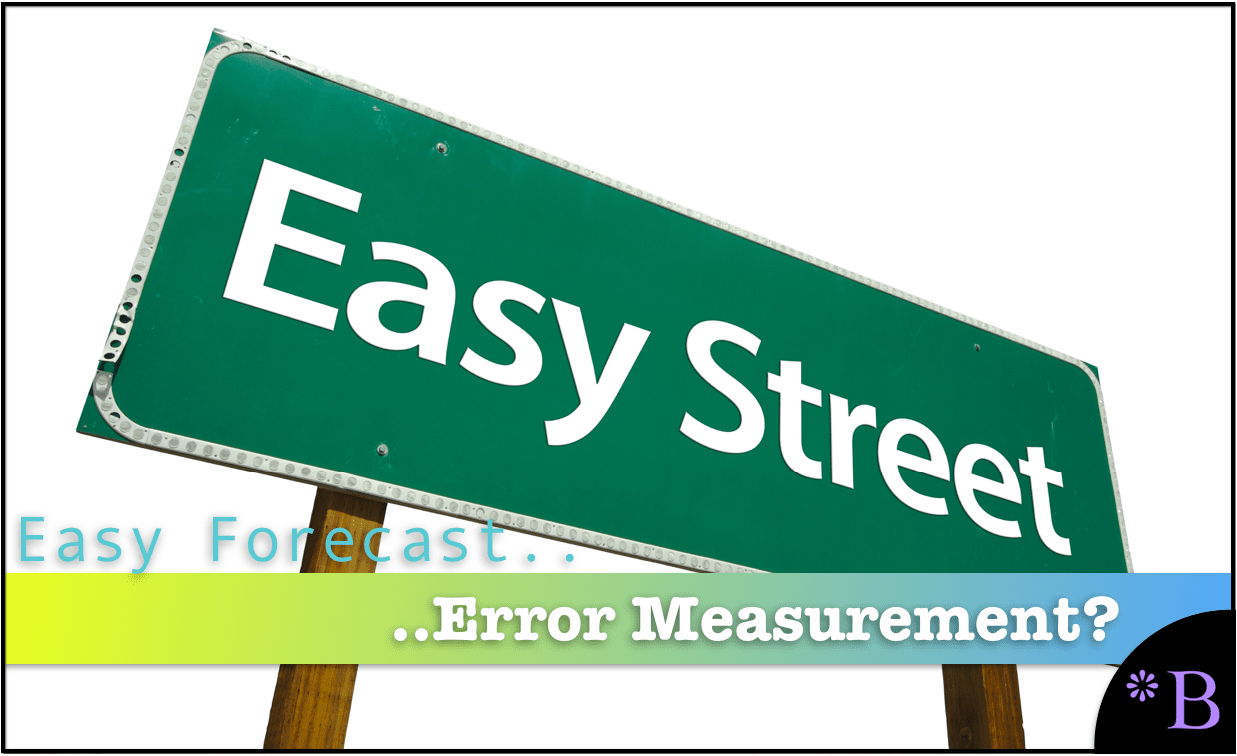# How Can Forecast Error be Calculated?

## Executive Summary

• Several universally accepted methods calculates the forecast error.
• After going over them, we will question if these methods are effective.## Text Introduction (Skip if You Watched the Video)

The question of how can forecast error be calculated is a common question in forecasting. We go through all of the major and even some of the minor methods of forecast error measurement. The calculation of these methods is widely known but not as well understood as generally thought. This article is written from the vantage point of working on forecasting projects for over a decade and a half and explaining forecast error calculation and measurement to many people of different levels in organizations and people in many different departments. You will learn the reality of forecast error calculation.

If you want to see our references for this article and related Brightwork articles, visit this link.

## How Can Calculate Forecast Error be Calculated

The most commonly used forecast error calculation methods are listed below.

We have not conducted a poll to determine the forecast error calculation method frequency, but having worked in the field for a while, this is our rough estimate of the relative frequency of use from most to least used.

To see an explanation of each, click the link.

1. MAPE: Mean Absolute Percentage Error
3. MAE: Mean Absolute Error
4. RMSE: Root Mean Square Error
5. MASE: Mean Absolute Scaled Error
6. sMAPE: Symmetrical MAPE

Something to notice is that the lesser-used forecast error calculations are lesser used as one goes down the list. A significant issue is forecast error measurements that are not proportional. Forecast error calculation methods that are not proportional are unintuitive and, hence difficult to understand.

## Where Does the Discussion of Forecast Error Tend to Stop?

When forecast error is discussed in most cases, the topic does not move beyond the item’s forecast error, generally at a location, what we call the product location combination. Supply chain planning cares about the forecast error at the product location combination as it hypothetically drives the safety stock.

## Aggregated Forecast Errors

In many cases, forecasts are created at a much more aggregated level, such as sales forecasting. However, when the forecast error is created at the product location, a significant error measurement element is how the error is reported outside of the individuals actually performing the forecasting. And this gets into the topic of both forecast error reporting and aggregation.

## How Many Dimensions to the Forecast Error?

The topic of forecast error tends to focus overwhelmingly on the forecast error measurement.

In fact, this is only one dimension of the forecast error measurement, as we cover in the article How is Forecast Error Measured in All of the Dimensions in Reality?

## Are the Common Forecast Error Measurements Effective?

Standard forecast error measurement calculations are used around the world. However, we have accumulated significant evidence that the standard or common forecast error measurement calculations are not effective at improving forecast accuracy. Our preference is MAPE, which is the most widely used forecast error measurement calculation of the standard forecast error measurement calculations. However, several years ago, we stopped using it.

• MAPE does not calculate with a zero in the denominator (so it is useless for calculating the error of low volume or lumpy demand history items).
• MAPE is also a bit complicated to weigh as one must calculate a very small weighed MAPE at the line or SKU level to have an accurate overall or total weighted MAPE value.

## Why Do the Standard Forecast Error Calculations Make Forecast Improvement So Complicated and Difficult?

It is important to understand forecasting error, but the problem is that the standard forecast error calculation methods do not provide this good understanding. In part, they don't let tell companies that forecast how to make improvements. If the standard forecast measurement calculations did, it would be far more straightforward and companies would have a far easier time performing forecast error measurement calculation.

## What the Forecast Error Calculation and System Should Be Able to Do

One would be able to for example:

1. Measure forecast error
2. Compare forecast error (For all the forecasts at the company)
3. To sort the product location combinations based on which product locations lost or gained forecast accuracy from other forecasts.
4. To be able to measure any forecast against the baseline statistical forecast.
5. To weigh the forecast error (so progress for the overall product database can be tracked)

##Getting to a Better Forecast Error Measurement Capability

A primary reason these things can not be accomplished with the standard forecast error measurements is that they are unnecessarily complicated, and forecasting applications that companies buy are focused on generating forecasts, not on measuring forecast error outside of one product location combination at a time. After observing ineffective and non-comparative forecast error measurements at so many companies, we developed, in part, a purpose-built forecast error application called the Brightwork Explorer to meet these requirements.

Few companies will ever use our Brightwork Explorer or have us use it for them. However, the lessons from the approach followed in requirements development for forecast error measurement are important for anyone who wants to improve forecast accuracy.